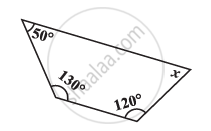# Find the Angle Measure X in the Given Figure - 1 - Mathematics

Find the angle measure x in the given Figure#### Solution

Sum of the measures of all interior angles of a quadrilateral is 360º. Therefore, in the given quadrilateral,

50° + 130° + 120° + x = 360°

300° + x = 360°

x = 60°

Concept: Angle Sum Property of a Quadrilateral
Is there an error in this question or solution?

#### APPEARS IN

NCERT Class 8 Maths